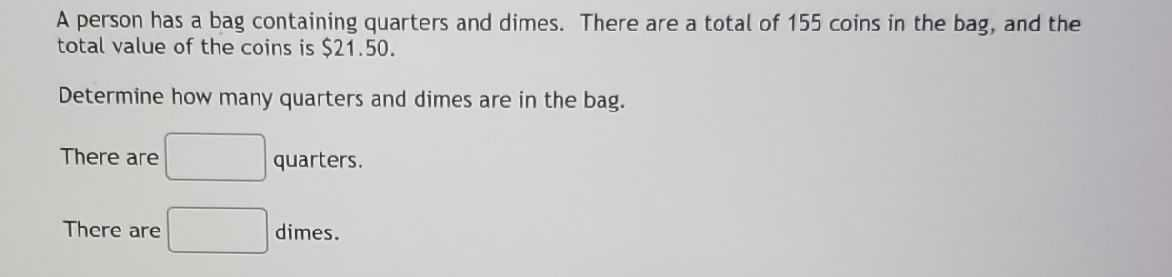### ¿Todavía tienes preguntas de matemáticas?

Pregunte a nuestros tutores expertos
Algebra
PreguntaA person has a bag containing quarters and dimes. There are a total of $$155$$ coins in the bag, and the total value of the coins is $$\ 21.50$$ . Determine how many quarters and dimes are in the bag. There are There are quarters.

$$d + q = 155,0.25 q + 0.1 d = 21.5 : q = 40 , d = 115$$

There are 40 quarters

There are 115 dimes

Steps

$$\left[ \begin{array} { c } { d + q = 155 } \\ { 0.25 q + 0.1 d = 21.5 } \end{array} \right]$$ Isolate $$d$$ for $$d + q = 155 : d = 155 - q$$

Substitute $$d = 155 - q$$

$$[ 0.25 q + 0.1 ( 155 - q ) = 21.5 ]$$ Simplify

$$[ 0.15 q + 15.5 = 21.5 ]$$

Isolate $$q$$ for $$0.15 q + 15.5 = 21.5 : q = 40$$ For $$d = 155 - q$$

Substitute $$q = 40$$

$$d = 155 - 40$$

Simplify

$$d = 115$$

The solutions to the system of equations are:

$$q = 40 , d = 115$$

Solución
View full explanation on CameraMath App.